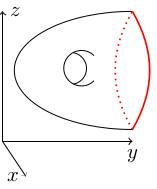# 3.02 Quotient topology: continuous maps

Below the video you will find accompanying notes and some pre-class questions.

# Notes

## Maps from a quotient space

(0.14) Suppose that $$X$$ is a space, $$\sim$$ is an equivalence relation on $$X$$, and $$Y$$ is another space. Given a map $$F\colon X\to Y$$ we say $$F$$ descends to the quotient if there exists a map $$\bar{F}\colon X/\sim\to Y$$ such that $$F=\bar{F}\circ q$$, where $$q\colon X\to X/\sim$$ is the quotient map.

(1.47) $$F$$ descends to the quotient if and only if $$F(x)$$ depends on $$x$$ only through its equivalence class $$[x]$$, that is if and only if $x_1\sim x_2\Rightarrow F(x_1)=F(x_2).$

(3.07) Conversely, given a map $$G\colon X/\sim\to Y$$, we can precompose with $$q$$ to get a map $$F:=G\circ q\colon X\to Y$$. In other words, $$\bar{F}=G$$. This means that:

Functions on the quotient space $$X/\sim$$ are in bijection with functions on $$X$$ which descend to the quotient.

## Continuity of maps from a quotient space

(4.30) Given a continuous map $$F\colon X\to Y$$ which descends to the quotient, the corresponding map $$\bar{F}\colon X/\sim\to Y$$ is continuous with respect to the quotient topology on $$X/\sim$$. Conversely, given a continuous map $$G\colon X/\sim\to Y$$, the composition $$F=G\circ q\colon X\to Y$$ is continuous and descends to the map $$\bar{F}=G$$ on the quotient.
(6.48) For the converse, if $$G$$ is continuous then $$F=G\circ q$$ is continuous because $$q$$ is continuous and compositions of continuous maps are continuous.

(7.33) If $$F$$ is a continuous map which descends to the quotient then, given an open set $$V\subset Y$$, the preimage $$\bar{F}^{-1}(V)$$ is open in the quotient topology on $$X/\sim$$ if and only if $$q^{-1}(\bar{F}(V))$$ is open in $$X$$ (by definition of the quotient topology). But $q^{-1}(\bar{F}^{-1}(V))=(\bar{F}\circ q)^{-1}(V)=F^{-1}(V)$ since $$F=\bar{F}\circ q$$. But $$F^{-1}(V)$$ is open because $$F$$ is continuous.

This will be extremely useful in future: to specify a continuous map on a quotient space $$X/\sim$$, we just need to specify a continuous map on $$X$$ and check it descends to the quotient.

# Pre-class questions

1. Let $$X$$ be the space in the figure below (thought of as sitting inside $$\mathbf{R}^3$$) and let $$A$$ be the red subset. Which of the following functions $$X\to\mathbf{R}$$ descends to the quotient $$X/A$$?
• the projection to the $$z$$-axis,
• the projection to the $$x$$-axis,
• the projection to the $$y$$-axis?